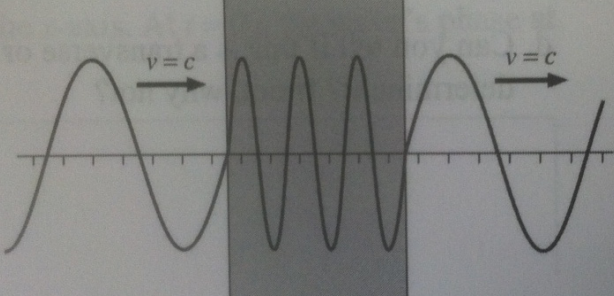# Problem: A light wave travels from vacuum through a transparent material and back to vacuum as shown in the figure. What is the index of refraction of this material?

###### FREE Expert Solution

Refractive index is defined by:

$\overline{){\mathbf{\eta }}{\mathbf{=}}\frac{\mathbf{c}}{\mathbf{v}}}$, where c is the speed of light in a vacuum and v is the speed of light in a medium.

In this diagram, the frequency does not change.

79% (211 ratings)###### Problem Details

A light wave travels from vacuum through a transparent material and back to vacuum as shown in the figure. What is the index of refraction of this material?